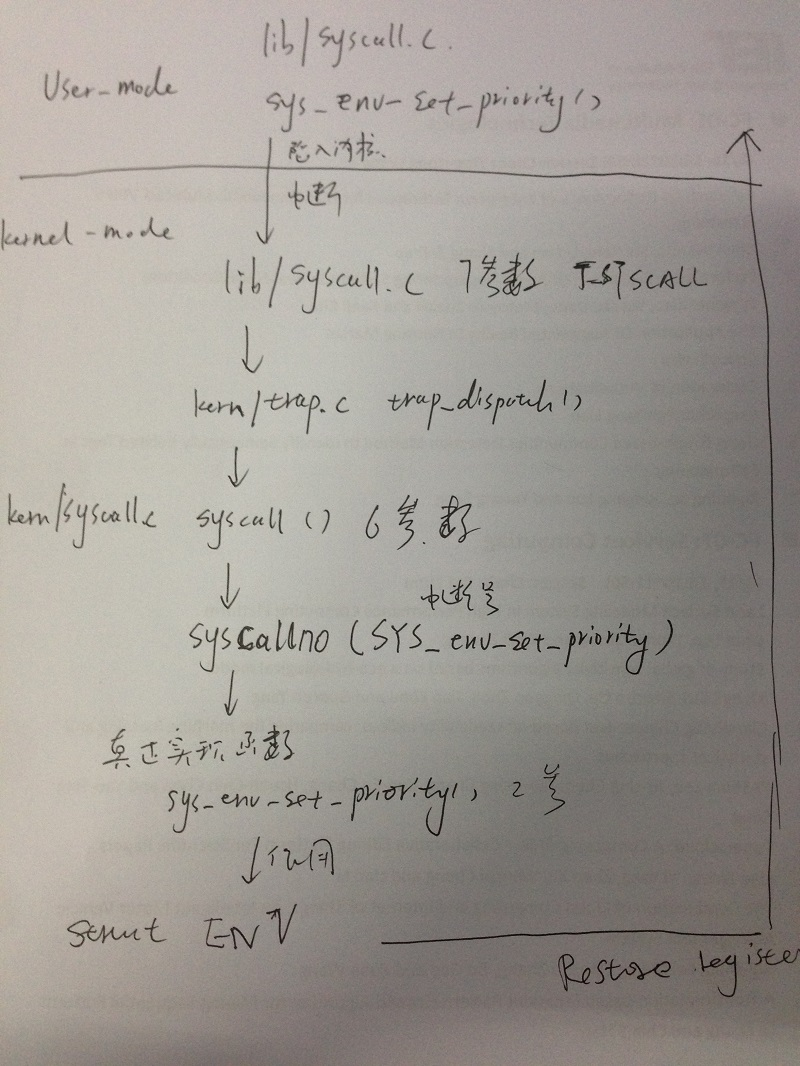# 在JOS上实现基于Priority的RR_sched

JOS在一开始实现的是简单的RR算法，没有优先级调度。

• 在inc/syscall.h中添加新的中断号：SYS_env_set_priority。

• 在kern/syscall.c的syscall()函数中添加相应switch分发语句。

• 在lib/syscall.c中添加供用户调用系统调用的库函数。

``````
int sys_env_set_priority(envid_t envid, int priority)
{
return syscall(SYS_env_set_priority, 1, envid, priority, 0, 0, 0);
}
``````

``````
void RR_Priority_sched(void)
{
int now_env,i;
if(curenv)
{
now_env = (ENVX(curenv->env_id) +1) % NENV;
}
else
{
now_env = 0 ;
}

uint32_t max_priority = 0;
int select_env = -1;

//  cprintf("NENV=%d\n",NENV);
for(i= 0;i< NENV;i++ , now_env = (now_env+1)%NENV)
{
if(envs[now_env].env_status ==ENV_RUNNABLE && (envs[now_env].env_priority > max_priority
||select_env == -1))
{
select_env=now_env;
max_priority = envs[now_env].env_priority;
cprintf ("I am CPU %d , I am in sched yield , I find ENV %d,Priority 0x%x, i = %d\n",
thiscpu ->cpu_id , select_env,max_priority,i);
}
}

//cprintf ("I am CPU %d , I am in sched yield , I find ENV %d,Priority %d\n",thiscpu ->cpu_id , select_env,max_priority);

if (select_env >= 0 && (! curenv || curenv ->env_status != ENV_RUNNING ||
max_priority >= curenv ->env_priority))
{
env_run (& envs[select_env ]);
}
if (curenv && curenv ->env_status == ENV_RUNNING)
{
env_run(curenv);
}

}
``````